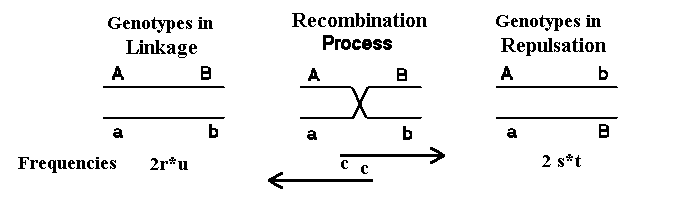### 2.5 Linkage, Calculation of gamete and genotype frequencies generation after generation

- Put your observed gamete frequencies or click the initiate Mendel button. If the mothers gamete frequencies sum to zero, the frequencies for the fathers is also applied for the mothers.
- Click on Next generation button calculate the gamete and genotype frequencies in next generation.

 Recombination freq -> Generation -> Set digit for print ->
Generation disequlibrium           ........Disequilibrium over generations......

In all cells with initial values you can put data and to get the results press the Next generation button. Table values are rounded to the desired number of decimals.

Example:
When you look at segregation of genes at two loci the gametes that can be formed are shown below and symbols are given for the observed and expected frequencies, the deviation between the two has the symbol D for disequlibrium.
``` Gamete  Observed frequency   Expected frequency    Deviation
--------------------------------------------------------------
AB       r                 p(A)*p(B)             D
Ab       s                 p(A)*q(b)             -D
aB       t                 q(a)*p(B)             -D
ab       u                 q(a)*q(b)             D```

The disequilibrium gets the symbol D can be calculated from the table above, and can also be calculated as the half of the difference in the frequency of double heterozygotes in linkage- and repulsion phase:

```      D = r*u - t*s    (= [f(AB/ab) - f(Ab/aB)]/2) from the table above
```
The maximum disequilibrium occurs, when all double heterozygotes are in linkage phase f(AB/ab) or in repulsion phase f(Ab/aB). See the Figure below.Are the recombination frequency c would D be reduced to D*(1-c) per generation, and for the n'th generation Dn = D0*(1-c)n.

If you apply the initial values r=0.1, s=0.2, t=0.3, u=0.4 and press the Next generation button, you will see calculated all the genotype frequencies and D=0.0199 rounded 0.02. If you continue using the Next generation button more times with c=0.5 you will see that D= approach 0 which means observed and expected gamete frequencies are equal i.e. r=0,12.

Questions:
Calculate the gamete frequency in the first 5 generations and the equilibrium gamete frequencies in the genetic system shown below where the recombination frequencies between the two loci A and B is c=0.2.

```Gamete  Observed frequency
AB        0.21
Ab 	  0.49
aB	  0.19
ab	  0.11
```

What would be the frequencies of the two types of double heterozygotes in next generation?
How many generations will it take to reach equilibrium within 0.02 units ? Repeat the calculations for c=0.05.

In a F3 after a cross between two pure lines aabb X AABB. What would be the gamete frequencies if c=.5 , .4 and .1 ?
Hints: Use the initiate mendel button and use the next generation button more times.

Back to theory, Back to theory (in Danish) or Back to the other programs